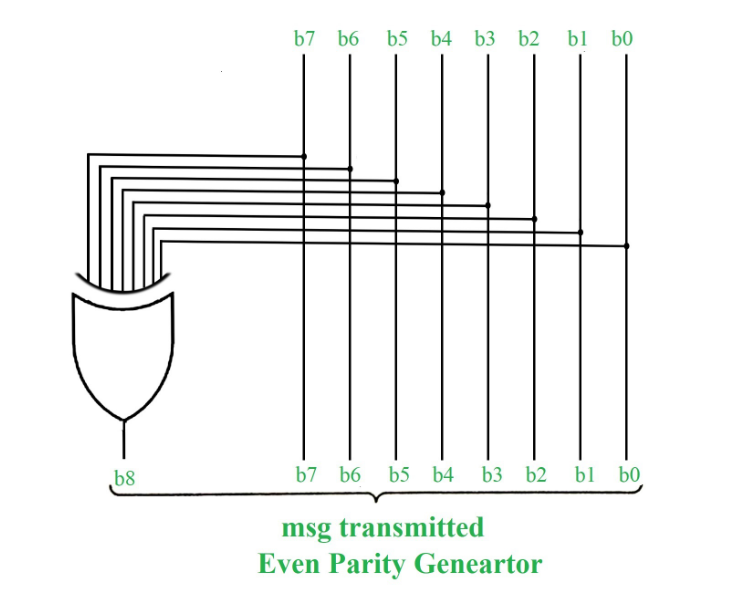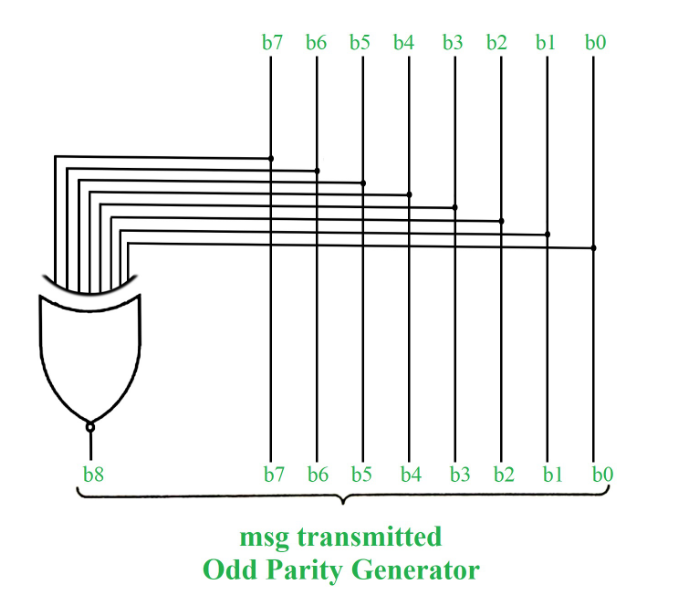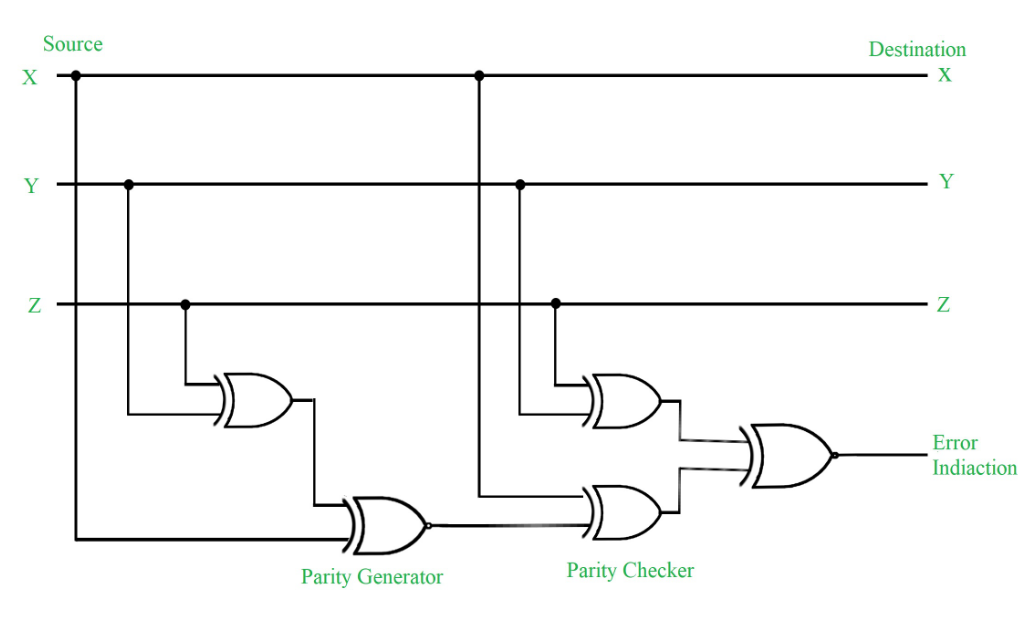# Error Detection Codes: Parity Bit Method

Error Detection Codes :
The binary information is transferred from one location to another location through some communication medium. The external noise can change bits from 1 to 0 or 0 to 1.This changes in values are called errors. For efficient data transfer, there should be an error detection and correction codes. An error detection code is a binary code that detects digital errors during transmission. A famous error detection code is a Parity Bit method.

Parity Bit Method :
A parity bit is an extra bit included in binary message to make total number of 1’s either odd or even. Parity word denotes number of 1’s in a binary string. There are two parity system-even and odd. In even parity system 1 is appended to binary string it there is an odd number of 1’s in string otherwise 0 is appended to make total even number of 1’s.In odd parity system, 1 is appended to binary string if there is even a number of 1’s to make an odd number of 1’s. The receiver knows that whether sender is an odd parity generator or even parity generator. Suppose if sender is an odd parity generator then there must be an odd number of 1’s in received binary string. If an error occurs to a single bit that is either bit is changed to 1 to 0 or O to 1, received binary bit will have an even number of 1’s which will indicate an error.The limitation of this method is that only error in a single bit would be identified.

Message (XYZ) P(Odd) P(Even)
000 1 0
001 0 1
010 0 1
011 1 0
100 0 1
101 1 0
110 1 0
111 0 1Figure – Error Detection with Odd Parity Bit

Points to Remember :

• In 1’s complement of signed number +0 and -0 has two different representation.
• The range of signed magnitude representation of an 8-bit number in which 1-bit is used as a signed bit as follows -27 to +27.
• Floating point number is said to be normalized if most significant digit of mantissa is one. For example, 6-bit binary number 001101 is normalized because of two leading 0’s.
• Booth algorithm that uses two n bit numbers for multiplication gives results in 2n bits.
• The booth algorithm uses 2’s complement representation of numbers and work for both positive and negative numbers.
• If k-bits are used to represent exponent then bits number = (2k-1) and range of exponent = – (2k-1 -1) to (2k-1).

Attention reader! Don’t stop learning now. Get hold of all the important CS Theory concepts for SDE interviews with the CS Theory Course at a student-friendly price and become industry ready.

My Personal Notes arrow_drop_upCheck out this Author's contributed articles.

If you like GeeksforGeeks and would like to contribute, you can also write an article using contribute.geeksforgeeks.org or mail your article to contribute@geeksforgeeks.org. See your article appearing on the GeeksforGeeks main page and help other Geeks.

Please Improve this article if you find anything incorrect by clicking on the "Improve Article" button below.